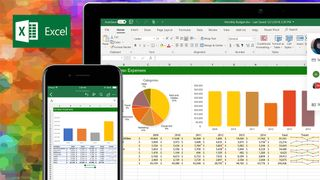# How to use VLOOKUP in ExcelMicrosoft Excel 2016 boasts a huge number of handy functions and utilities, many of which go untouched by the average user. If you find yourself repeatedly consulting the same table to find data, however, have a gander at VLOOKUP. Short for "vertical lookup," VLOOKUP takes advantage of vertically-aligned tables to quickly locate data associated with a given value.

Note: though this tutorial was written for Microsoft Excel 2013, it works the same way in the latest version of Excel.

If you know the name of a product, for instance, and you want to quickly determine its price, simply enter the product name into Excel and VLOOKUP will find the price for you. To the novice Excel user, setting up VLOOKUP can look like an intimidating process — but it needn't be. Just follow our step-by-step tutorial on how to use VLOOKUP in Excel today.

## How to use VLOOKUP in Excel

1. Click the cell where you want the VLOOKUP formula to be calculated.Click the cell where you want the VLOOKUP formula to be calculated.

2. Click Formulas at the top of the screen.

3. Click Lookup & Reference on the Ribbon.

4. Click VLOOKUP at the bottom of the drop-down menu.

5. Specify the cell in which you will enter the value whose data you're looking for. In this case, our lookup value is H2, since this is where we will input the name of a tournament such as "PGA Championship," so we input "H2" in the lookup_value box of the popup window. Once we've set up VLOOKUP properly, Excel will return the tournament's Total Rating Value in cell H3 when we type the tournament name in cell H2.Specify the cell in which you will enter the value whose data you're looking for.

6. Specify the data that you want VLOOKUP to use for its search in the table_array box. In this case, we've selected the entire table (excluding the headers).Specify the data which you want VLOOKUP to use for its search in the table_array box.

7. Specify the column number which VLOOKUP will use to find the relevant data in the col_index_num box. Somewhat confusingly, VLOOKUP requires you to use the numerical value of the column rather than its letter value. In this case, we want VLOOKUP to use the Total Rating Value column — column D — so we enter the number 4.Specify the column number that VLOOKUP will use to find the relevant data in the col_index_num box.

8. Specify whether you need an exact match by entering either FALSE (exact match) or TRUE (approximate match) in the range_lookup box. In this case, we want an exact match so we enter FALSE.Specify whether you need an exact match by entering either FALSE (exact match) or TRUE (approximate match) in the range_lookup box.

9. Click OK at the bottom of the popup window.

10. Enter the value whose data you're searching for. In our example, we want to find the Total Value Rating of the PGA Championship, so we type "PGA Championship" into cell H2 and VLOOKUP automatically produces the Total Value Rating (in this case, 914) in cell H3.